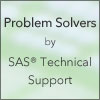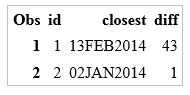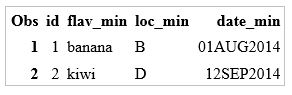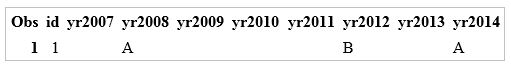After you start to use arrays, it is easy to see their usefulness and power. In this post, I will share tips andtechniques that can increase the functionality of arrays and enable you to use programming shortcuts to take maximum advantage of them.

These tips will also streamline your programming, even if you are not using arrays. I'll provide complete code examples for each tip at the end of each section, as well as explain how the code is constructed along the way.

## Use arrays to zoom out for greater perspective

One of the biggest uses of arrays, of course, is to reshape your data from one observation per identifying variable per data point, to one observation per ID containing all the data points for that ID. For example, consider the following data set:

Now, you want the values of the EVENT variable to become the names of the variables in the output data set, as shown here:

How do you know which variables to create if your data set is more complicated than shown above?

## Create a macro variable

You can use the SQL procedure to create a macro variable (called EVENTLIST here) that contains all the unique values of EVENT. You then can use that macro variable in your array definition statement:

```proc sql noprint; select distinct event into :eventlist separated by ' ' from work.events; quit;```

When you run the following statement, the resulting log shows that &EVENTLIST contains the unique values for the EVENT variable:

%put &eventlist;

3365 %put &eventlist;
aaa bbb ccc ddd

You can then use the EVENTLIST macro variable in an ARRAY statement to define the variables that you are including:

array events_[*] &eventlist;

## Use CALL MISSING to set all variables

However, you do not want the values for the previous ID to contaminate the next ID, so you need to reset the array variables to missing with each new ID. You can use a DO loop that uses FIRST.variable and LAST.variable processing in order to set each value to missing:

```if first.id then do; do i = 1 to dim(events_); events_[i] = .; end; end;```

DO loops were commonly used in the past to initialize all elements of an array to missing. And it still needs to be used if you want to set all the elements to a non-missing value, such as 0. However, you can replace this code with one function call. The CALL MISSING routine sets all the variables in the array to missing in one statement:

if first.id then call missing(of events_[*]);

## Compare via OF variable-list syntax

The OF variable-list syntax is another helpful feature. You can use OF with a list of variables or an array reference.

So, now you have the EVENT values as variable names. How are you going to compare the value of a variable in an observation with a variable name in the array? You can reference each element of the array that you created with EVENTS_[i], but that will return the value of that element. For this comparison, you need to obtain the variable name for each element in the array.

To return the name of each variable in the array, you can use the VNAME function:

if event = vname(events_[i]) then do;

Now you can find when the value of the EVENT variable in the original data set matches the name of the variable in the array.

## Additional ways to extract variables information in arrays

Other variable information functions can also be used in the same way to extract information about each of the variables in the array.

In this example, a value that is read from each observation can match only one variable name in the array, so you want to stop when you achieve that instead of continuing the DO loop.

To stop the DO loop at that point, use the LEAVE statement, which stops processing the current loop and resumes with the next statement in the DATA step.

Because you want to output only one observation per ID, you must explicitly use the OUTPUT statement at the end of each ID to override the default output that occurs at the end of each iteration of the DATA step:

if last.id then output;

## Combine above methods with DATA Step

Putting all these methods together, here is a short DATA Step that will reshape the original data set into the output data set that you want:

```data events_out (drop =i event); set events; by id; array events_[*] &eventlist; retain &eventlist; if first.id then call missing(of events_[*]); do i = 1 to dim(events_); if event = vname(events_[i]) then do; events_[i] = 1; leave; end; end; if last.id then output; run;```

## Bonus shortcuts using arrays

Want to use even more shortcuts with arrays? Here, your original data has a numeric variable called RATING, which is included in every observation:

You want to find the lowest rating and corresponding event and also the highest rating and corresponding event for each ID. You can start off by defining the arrays and reading each observation in the same way as in the previous example. In this case, you have two arrays, so you need to read both the EVENTS and RATINGS variables into their own arrays:

array ratings_;
array events_ \$3.;
…more SAS statements…
ratings_[count]=rating;
events_[count]=event;

After you have read all the observations for an ID, how do you find the lowest rating? Of course, you can use a DO loop to loop through the array and check each value to see whether it is the lowest and keep track of which one it is. Or you can use the OF array syntax with the MIN and MAX functions:

lowest_rating=min(of ratings_[*]);
highest_rating=max(of ratings_[*]);

This code returns the value of the lowest and highest ratings for ID 1, which are 3 and 9, respectively. But you also want to know which events were rated the highest and lowest for this ID. First, you have to determine which elements in the RATINGS_ array had the lowest and highest values. You know the values, but you do not yet know which specific elements match those values. Use the WHICHN function to determine the indexes for those values in the array. Keep in mind that if there is more than one element that matches the value using the WHICHN function, the function returns only the index of the first element in the array to match:

min_index=whichn(lowest_rating, of ratings_[*]);
max_index=whichn(highest_rating, of ratings_[*]);

For ID 1, these functions return 3 and 5, respectively. Now you can use the indexes to retrieve the corresponding elements in the EVENTS_ array:

lowest_rated_event=events_[min_index];
highest_rated_event=events_[max_index];

Putting all these methods together, here is the complete code for returning the desired results:

```data rating (keep = id lowest_rated_event lowest_rating highest_rated_event highest_rating); set events; by id; array ratings_; array events_ \$3.; retain ratings: events:; if first.id then call missing(of ratings_[*],of events_[*], count); count + 1; ratings_[count] = rating; events_[count]=event; if last.id then do; lowest_rating = min(of ratings_[*]); highest_rating = max(of ratings_[*]); min_index =whichn(lowest_rating,of ratings_[*]); max_index=whichn(highest_rating, of ratings_[*]); lowest_rated_event=events_[min_index]; highest_rated_event=events_[max_index]; output; end; run;```

Here is the resulting output:

You can expand the functionality and capability of arrays by incorporating other features of the SAS programming language in your DATA Step code. I hope these tips and links improve your array use and lead you to explore even more ways to work with arrays. The resource below complements this post and provides additional tips and tricks.

Adventures in Arrays | Learn more about array processing!Arrays are a powerful SAS programming tool. They can be used to simplify coding for repetitive calculations, to transpose data and to evaluate variables in a non-sequential manner. Sometimes users are intimidated by the term array, but in SAS, an array is simply a grouping of variables that lasts for the duration of the DATA step where the array is defined.

In this blog post, I’ll provide the code and explanations for using SAS arrays to solve these common programming problems:

• Find the closest value to a constant value in a group of variables.
• Determine which variable in a group meets certain criteria and report corresponding variables.
• Transpose data and manipulate a group of values.

### Find the closest value in a group of variables to a constant value.

In this example, we want to know, for each value of ID, what was the closest visit date to 01Jan2014? By defining an array date with the variables visit1-visit5, we can use a DO loop to evaluate each visit date to see whether it is closer than the previous ones. If so, we redefine the closest and diff variables to contain the closest visit date and the difference between that date and 01Jan2014.

When variables are listed in the array definition statement, SAS first looks for existing variables with those names. If they do not exist, SAS creates them. The default for variables created in an array is numeric type, as it is for variables created otherwise.

The dim() function returns the dimension of an array. You can use this in place of hardcoding the end value.

The string '01jan2014'd is a date constant and is the syntax to refer to a specific date.

```data visits;
input id (visit1-visit5) (:date9.);
datalines;
1 11aug2012 14sep2014 04nov2013 13feb2014 30jun2013
2 23aug2014 02jan2014 01jan2012 08nov2014 15may2013
run;

data results (keep = id closest diff);
format id 8. visit1-visit5 closest date9.;
set visits;
array date[*] visit1-visit5;
closest = date;
diff = abs(date - '01jan2014'd);
do i = 2 to dim(date);
if abs(date[i] - '01jan2014'd) < diff then do;
closest = date[i];
diff = abs(date[i] - '01jan2014'd);
end;
end;
run;

proc print; run;
```

For each ID, the date closest to 01Jan2014 and the difference in number of days is displayed.### What variable in a group meets a certain criteria, and what are the corresponding variables?

In this example, we want to know, for each respondent ID, which flavor received the lowest score and what was the date and location of that score?

The definition for the flav array is using a name range list to refer to all variables in order between apple and kiwi inclusive.

We can use a special SAS name list like _character_ to refer to all character variables currently defined in the DATA step. The LENGTH statement for a new character variable flav_min has to be after the array definition statement if we do not want it included in the array of character variables.

Since the variables for the date array are not listed, SAS first looks for, and if needed, creates variables with the names date1-date5.

The OF operator can be used with certain functions to perform that function on the variable list or array specified.

Once we know the minimum value, we loop through the variables to determine which element matches the minimum value. Then we use the vname() function to return the name of the variable. We find the corresponding variables in the loc and date arrays by using the same i subscript to obtain those values.

The LEAVE statement stops processing the current loop. We do not need to continue processing when we have found the value that matches the minimum. (In this case, we are assuming that there is only one value that matches the minimum.)

```data flavors;
input id (apple orange banana grape kiwi) (:8.) (loc1-loc5) (:\$1.) (date1-date5) (:date9.);
datalines;
1 100 98 75 84 92 A A B C D 10jul2014 12jul2014 01aug2014 10aug2014 12sep2014
2 80 78 83 88 72 B C A E D 10jul2014 12jul2014 01aug2014 10aug2014 12sep2014
;

data flavor_min (keep = id flav_min date_min loc_min);
retain id flav_min loc_min date_min;
format date_min date9.;
set flavors;
array flav apple--kiwi;
array loc _character_;
array date;
length flav_min \$10;
minflav = min(OF flav[*]);
do i = 1 to dim(flav);
if minflav = flav[i] then do;
flav_min = vname(flav[i]);
date_min = date[i];
loc_min = loc[i];
leave;
end;
end;
run;

proc print; run;```

For each ID, the resulting output displays the flavor with the lowest score and the corresponding location and date where that score occurred.### Transpose data and manipulate a group of values.

In this example, for each ID, we want to keep only the last value of each identical series and set the remaining values to missing so that there is only one observation per ID in the output. In this case, the data is originally what is sometimes called “long”, that is, many observations per BY group.  We want to make it “wide”, or one observation per BY group.

Instead of using the default lower and upper bounds for the array, we specify the array bounds 2007:2014. That way, we can use the value of year to indicate which array element should be updated.

If we do not specify the variable names in the array, they will be defined yr1-yr7 even though the bounds of the array have been defined differently. We explicitly name them yr2007-yr2014 because those are the variable names we want. These are character variables, so we have to specify that in the array definition statement with a \$ after the array name.

We use the CALL MISSING() routine to set all the values of the array to missing at the start of each BY group.

Since we want to transpose the data, we read the value for each observation into an element of the array corresponding the year.  We can use the year value as the subscript for the array and set the value of the correct yrn variable to value.

Once we have read the entire BY group into the array, we’ll use a DO loop to check each value of the array against the next value.  If they are the same, we set the current one to missing. We do an explicit OUTPUT at the end of the DO loop because we don’t want to do the default output at the end of each iteration of the data set. We just want to output one observation for the entire BY group.

```data years;
input id year value \$1.;
datalines;
1 2007 A
1 2008 A
1 2010 B
1 2011 B
1 2012 B
1 2013 A
1 2014 A
run;

data years_out (drop = year i value);
set years;
by id;
array yr[2007:2014] \$ yr2007-yr2014;
retain yr:;
if first.id then do;
call missing (of yr[*]);
end;
yr[year] = value;
if last.id then do;
do i = 2007 to 2013;
if yr[i] = yr[i+1] then yr[i] = '';
end;
output;
end;
run;

proc print; run;```

The output data set has only one observation for each ID with repeated values set to missing until the last one in a series.### Just getting started with arrays?

The best place to start looking for information about arrays is the section on Array Processing in the Language Reference: Concepts document.

There are more examples in the  Statements: Reference document under ARRAY statement and Array Reference Statement.